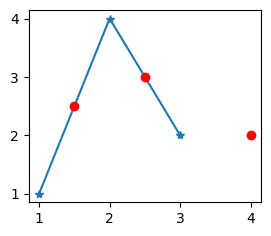search
Search
Unlock 100+ guides
search toc
close
Cancel
Post
account_circle
Profile
exit_to_app
Sign out
search
keyboard_voice
close
Searching Tips
Search for a recipe:
"Creating a table in MySQL"
Search for an API documentation: "@append"
Search for code: "!dataframe"
Apply a tag filter: "#python"
Useful Shortcuts
/ to open search panel
Esc to close search panel
to navigate between search results
d to clear all current filters
Enter to expand content previewDoc SearchCode Search BetaSORRY NOTHING FOUND!
mic
Start speaking...Voice search is only supported in Safari and Chrome.
Shrink
Navigate to

# NumPy | interp method

schedule Aug 12, 2023
Last updated
local_offer
PythonNumPy
Tags
expand_more
mode_heat
Master the mathematics behind data science with 100+ top-tier guides
Start your free 7-days trial now!

Numpy's `interp(~)` method computes the linear interpolated values given data points.

# Parameters

1. `a` | `array-like`

The x-values you want to evaluate at.

2. `xp` | `array-like`

A one-dimensional array of x-values.

3. `fp` | `array-like`

A one-dimensional array of y-values.

4. `left` | `float` | `optional`

The value to return when a value in `a` is less than `xp`, that is, we're to the left of the bounds. By default, the first value of `fp` is returned.

5. `right` | `float` | `optional`

The value to return when a value in `a` is larger than `xp[len(xp)-1]`, that is, we're to the right of the bounds. By default, the last value of `fp` is returned.

# Return value

A Numpy array holding the y-values that have been linearly interpolated given the data points.

# Examples

## Basic usage

``` x_vals_to_evaluate = [1.5, 2.5, 4]x = [1,2,3]y = [1,4,2]interp_y = np.interp(x_vals_to_evaluate, x, y)# Let's graph the interpolated y-valuesplt.plot(x,y, marker="*")plt.plot(x_vals_to_evaluate, interp_y, "o", color="red")plt.show() ```

This gives us the following:Here, the stars are the data-points we used to construct the linear relationship. The red dots represent the data points that were interpolated at the specified `x=[1.5, 2.5, 4]`. The corresponding interpolated y-values are as follows:

``` interp_y array([2.5, 3. , 2. ]) ```

Finally, look at the red dot on the far right. We tried to interpolate at a x-value that was outside our specified x-bounds (`xp`), and by default, the returned y-value in this case is the last value of `fp` (2 in this case).

We could return an arbitrary value in this case by specifying the `right` parameter.

thumb_up
thumb_down
Comment
Citation
Ask a question or leave a feedback...
thumb_up
0
thumb_down
0
chat_bubble_outline
0
settings
Enjoy our search
Hit / to insta-search docs and recipes!